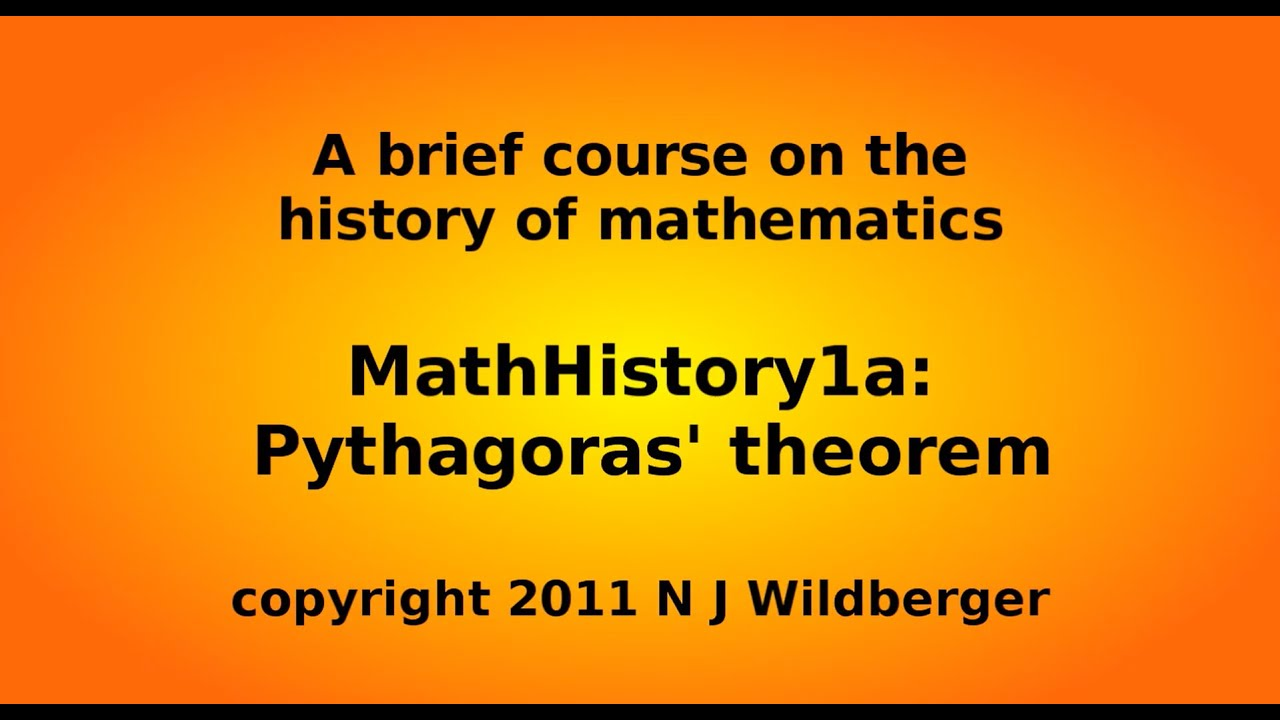# extra-math.min

Mathematics is the classification and study of all possible patterns. This package includes some common statistics functions. [(1)] :package: NPM, :smiley_cat: GitHub, :running: RunKit, :vhs: Asciinema, :moon: Minified, :scroll: Files, :newspaper: JSDoc, :blue_book: Wiki.

## Usage no npm install needed!

``````<script type="module">
import extraMathMin from 'https://cdn.skypack.dev/extra-math.min';
</script>``````

Mathematics is the classification and study of all possible patterns. This package includes some common statistics functions. (1)
:package: NPM, :smiley_cat: GitHub, :running: RunKit, :vhs: Asciinema, :moon: Minified, :scroll: Files, :newspaper: JSDoc, :blue_book: Wiki.

Stability: Experimental.

This is browserified, minified version of extra-math.
It is exported as global variable math.
CDN: unpkg, jsDelivr.

``````const math = require("extra-math");
// import * as math from "extra-math";
// import * as math from "https://unpkg.com/extra-math@1.1.15/index.mjs"; (deno)

math.sum(1, 2, 3, 4);
// 10

math.median(1, 7, 8);
// 7

math.variance(1, 2, 3, 4);
// 1.25

math.lcm(2, 3, 4);
// 12
``````

## Index

Method Action
sum Finds sum of numbers (Σ).
product Finds product of numbers (∏).
mean Finds average of numbers.
median Finds the value separating the higher and lower halves of numbers.
modes Finds the values that appear most often.
range Finds the difference between the largest and smallest values.
variance Finds the mean of squared deviation of numbers from its mean.
erf Finds error function value of number (approximation).
erfc Finds complementary error function value of number (approximation).
rem Finds remainder of x/y with sign of x (truncated division).
mod Finds remainder of x/y with sign of y (floored division).
modp Finds remainder of x/y with +ve sign (euclidean division).
gcd Finds greatest common divisor of numbers.
lcm Finds least common multiple of numbers.
binomial Finds ways to choose k elements from a set of n elements.
multinomial Finds ways to put n objects in m bins (n=sum(ki)).